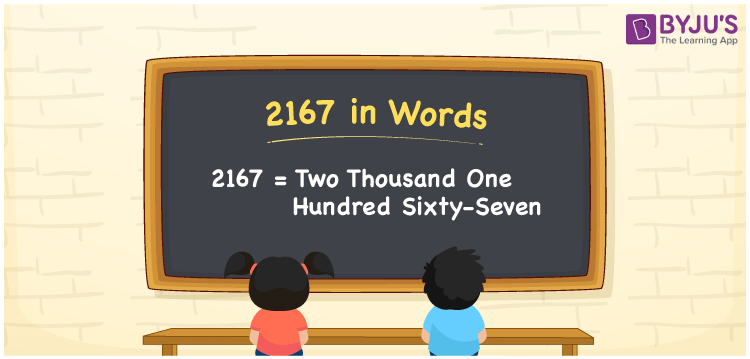# 2167 in Words

We can write 2167 in words as Two thousand one hundred sixty-seven. If you paid an amount of Rs. 2167 for envelopes, then you can say, “I paid Two thousand one hundred sixty-seven rupees for the envelopes”. That means we use the word form of the cardinal number 2167 to express or communicate the number to others. In this article, you will learn how to convert 2167 into words using a simple place value chart.

 2167 in words Two thousand one hundred sixty-seven Two thousand one hundred sixty-seven in Numbers 2167

## 2167 in English Words

Using the English alphabet, we generally write numbers in words. So, we can spell 2167 in English as “Two thousand one hundred sixty-seven”.## How to Write 2167 in Words?

Let’s learn how to write the number 2167 in words using a four-column place value chart given below since 2167 is a four-digit number.

 Thousands Hundreds Tens Ones 2 1 6 7

Here, ones = 7, tens = 6, hundreds = 1, and thousands = 2.

The above digits can be expanded as,

2 × Thousand + 1 × Hundred + 6 × Ten + 7 × One

= 2 × 1000 + 1 × 100 + 6 × 10 + 7 × 1

= 2000 + 100 + 60 + 7

= Two thousand + One hundred + Sixty + Seven

= Two thousand one hundred sixty-seven

Therefore, 2167 in words = Two thousand one hundred sixty-seven.

As we know, 2167 is a natural number that precedes 2168 and succeeds 2166.

2167 in words – Two thousand one hundred sixty-seven

Is 2167 an even number? – No

Is 2167 an odd number? – Yes

Is 2167 a prime number? – No

Is 2167 a composite number? – Yes

Is 2167 a perfect square number? – No

Is 2167 a perfect cube number? – No

## Frequently Asked Questions on 2167 in Words

Q1

### What number is Two thousand one hundred sixty-seven in words?

The number 2167 is expressed as Two thousand one hundred sixty-seven in words.
Q2

### How to write Rs. 2167 in words on a cheque?

On a cheque, we generally write Rs. 2167 in words as “Two thousand one hundred sixty-seven rupees only”.
Q3

### Write 2167.8 in words.

We can write 2167.8 in words as Two thousand one hundred sixty-seven point eight.